## Apollonius Circles

There are two completely different definitions of the so-called Apollonius circles:

1. The set of all points whose distances from two fixed points are in a constant ratio(Ogilvy 1990).

2. The eight Circles (two of which are nondegenerate) which solve Apollonius' Problem for three Circles.

Given one side of a Triangle and the ratio of the lengths of the other two sides, the Locus of the third Vertex is the Apollonius circle (of the first type) whose Center is on the extension of the given side. For a given Triangle, there are three circles of Apollonius.

Denote the three Apollonius circles (of the first type) of a Triangle by,, and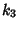, and their centers,, and. The centeris the intersection of the side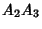with the tangent to the Circumcircle at.is also the pole of the Symmedian Pointwith respect to Circumcircle. The centers,, andare Collinear on the Polar ofwith regard to its Circumcircle, called the Lemoine Line. The circle of Apolloniusis also the locus of a point whose Pedal Triangle is Isosceles such that.Let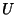andbe points on the side lineof a Triangle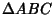met by the interior and exterior Angle Bisectors of Angles. The Circle with Diameter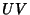is called the-Apollonian circle. Similarly, construct the- and-Apollonian circles. The Apollonian circles pass through the Vertices,, and, and through the two Isodynamic Pointsand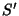. The Vertices of the D-Triangle lie on the respective Apollonius circles.

See also Apollonius' Problem, Apollonius Pursuit Problem, Casey's Theorem, Hart's Theorem, Isodynamic Points, Soddy Circles

References

Johnson, R. A. Modern Geometry: An Elementary Treatise on the Geometry of the Triangle and the Circle. Boston, MA: Houghton Mifflin, pp. 40 and 294-299, 1929.

Ogilvy, C. S. Excursions in Geometry. New York: Dover, pp. 14-23, 1990.